## Thursday, July 4, 2019

### Talk on KiwiSDR TDoA is available on YouTube

This is just a short note that my recent talk on KiwiSDR TDoA at the Software Defined Radio Academy 2019, which took place during the German Ham Convention in Friedrichshafen, Germany, is now available on YouTube.

For other talks from this meeting see http://youtube.sdra.io/.

## Tuesday, June 25, 2019

### 4800 symb/sec QPSK signal on 10160 kHz (2)

This in an update related to the last blog post. As before, all analysis is done in GNU octave.

In order to extract the bit stream from the signal on 10160 kHz we assume that it (D)QPSK modulated and use the gray code mapping [0,1,2,3] -> [0,1,3,2].

## Assuming QPSK modulation

The extracted bits are shown below in terms of 894-bit long frames.bit stream in terms of 24×41 frames (QPSK)

The rank-deficiency analysis of this bit stream indicates the presence of a convolutional code:rank-deficiency (QPSK)

For obtaining the rank-deficiencies above, the bit stream has been aligned by using an offset for which the R(k)/k is minimal, i.e., at 10+m×24:alignment of bit stream for the rank-deficiency analysis (QPSK)

As a result, the code rate is n/k=1/4, and the length of the dual code is 41. The latter is quite large and therefore hints at the use of some form of Turbo Code, or something similar to it.

## Assuming DQPSK modulation

The extracted bits are shown below in terms of 894-bit long frames. Unlike for QPSK modulation the bits in positions [1,18,19,24]+m×24 are the same in each frame.zoom of bit stream in terms of 24×41 frames (DQPSK)

Also assuming DQPSK modulation, the presence of a convolutional code is detected; it has code rate 7/12 and the length of the dual code is 41. (This might be a Turbo Code with 14 systematic bits and 5+5 parity bits)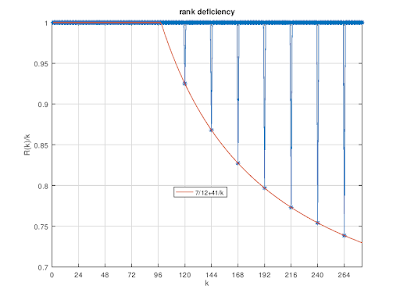rank deficiency (DQPSK)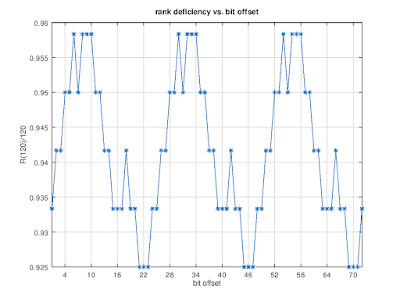alignment of bit stream for the rank-deficiency analysis (DQPSK)

## Summary

It is unlikely by coincidence that the frame length is 24×41, the length of the dual code is 41, and the distance between the rank deficiencies is 24.

The fixed bits in each frame when assuming DQPSK modulation are likely being used for frame synchronization: in this case, 4 of the 14 systematic bits are used for frame alignment and there are 5+5 parity bits.

Obviously it would be interesting to figure out the full parameters of the convolutional coding.

## Friday, June 14, 2019

### 4800 symb/sec QPSK signal on 10160 kHz

Currently there is a signal active on 10160 kHz. It is QPSK modulated with 4800 symb/sec. The autocorrelation function for the QPSK symbols has peaks at multiples of 492=12×41 symbols:symbol autocorrelation

Arranging the QPSK symbols in frames of 492 symbols, some interesting structures can be seen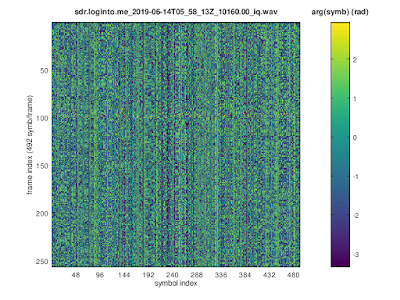492 symbols / frame

When the symbols are arranged in terms of 12-symbol long frames, some columns of the resulting matrix have a periodicity of 41 in the squared symbols.

TDoA geo-location indicates a location close to Luxembourg.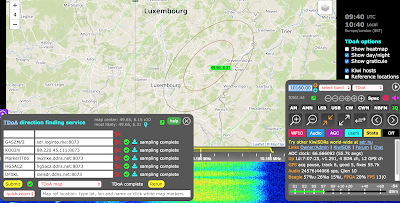TDoA

## Friday, May 31, 2019

### THALES Salamandre HFXL bursts

In the last days THALES Salamandre HFXL signals were again active, as was also noticed by Antonio.

TDoA points to the area around Paris. However, judging from the observed field strengths, there were transmitters at (at least) two locations active.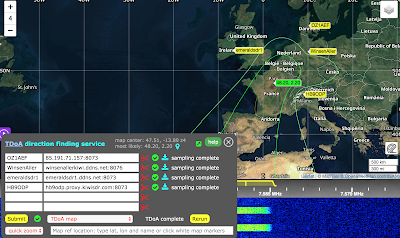TDoA

A 72-frame long HFXL burst is shown below (most bursts consist of 9 frames). The symbols were obtained by manually correcting for the doppler offset and searching for the correct symbol timing using GNU octave. The burst consists of frames with 287 symbols where the last 31 symbols are +-sign miniprobes.HFXL burst

The preamble consists of three parts where the 1st two parts are following MIL-STD-188-110 App. C and the 3rd part is a proprietary extension. It encodes 10 di-bits D0,...,D9 containing information about the used modulation schema and other signal parameters.HFXL preamble

It is known that the first few di-bits encode information about the used modulation schema:
[D0, D1, D2] = [(1,M0), (1,M1), (1,M2)]
In order to study these bursts more systematically the extended HFXL preamble was implemented in gr-digitalhf as an extension of the 110C mode. A number of recorded HFXL signals were analyzed and the constellations shown below were found (not shown: BPSK). Note that the BPSK and QPSK modes are scrambled to 8PSK symbols.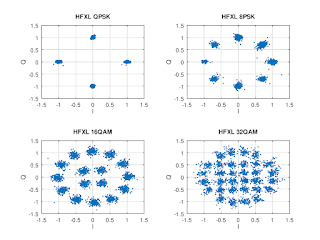HFXL constellations after descrambling

The table below shows a map between [M0,M1,M2] and the channel symbols after descrambling. 64QAM mode was not observed and is therefore missing in the table below.
 M0,M1,M2  Channel symbols after descrambling
0, 0, 0   BPSK
1, 0, 0   QPSK
0, 1, 0   8PSK
1, 1, 0  16QAM
0, 0, 1  32QAM
1, 0, 1   ----
0, 1, 1   QPSK chips with phases [+1,-1,-1,+1]
1, 1, 1   ----

At first is was puzzling that two combinations of [M0,M1,M2] correspond to QPSK descrambled symbols. Having a closer look at (0,1,1) bursts, it can be seen that it consists of QPSK symbols Si spread out using [+1,-1,-1,+1] chips, i.e.,
..., Si,-Si,-Si,Si, Si+1,-Si+1,-Si+1,Si+1, ...
making it a very robust mode to transmit data.QPSK (0,1,1) mode

The bits extracted from the 72-frame long burst shown above have a mean of 0.377; assuming that the probability for a bit to be 1 is 0.5 this translates into 24.6% of the payload consisting of zeros. If this is correct then one should see long strings of 0 bits.

Assuming that the 72 frames in this burst consist of two blocks of 36 frames, and that an interleaver of the form specified in MIL-STD-188-110 App. D is used, the maximum run lengths for all interleaver increments are shown below. For both interleaver blocks there is a maximum for an interleaver increment of 469.maximum run length as a function of interleaver increment

Assuming that the correct interleaver increment is 469, the deinterleaved bits are shown below:
• there are four gaps, each about 215 bits long
• the probability of a bit to be 1 if it is not in one of these gaps is 46.1% for the 1st block and 46.9% for the 2nd block
• the distribution of the deinterleaved bits for both blocks is similar and shows an interesting patterndeinterleaved bits for interleaver increment 469

As this analysis is based on a single burst with 72 frames, it might be a mere coincidence. In addition it is worth noticing that using the same interleaver increment, assuming that all 72 frames are a single interleaver block,  the double of the bit patterns above is obtained.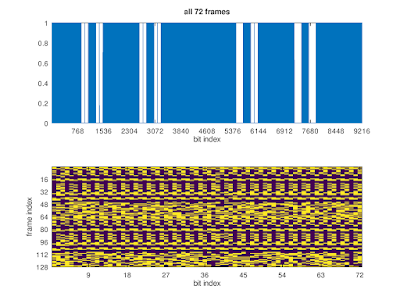deinterleaved bits for interleaver increment 469

## Wednesday, May 29, 2019

### MIL-STD-188-110 App.C QAM

Recently MIL-STD-188-110 App C  (STANAG 4539) signals were found on a KiwiSDR. This made it possible to complete the corresponding decoder in gr-digitalhf which includes an adaptive channel filter, descrambling, deinterleaving, and convolutional decoding. For the QAM modes descrambling is done by applying an XOR operation on the soft decisions.QAM32 constellation obtained after decoding with gr-digitalhf
Using the path metrics of the convolutional decoder it was verified that the whole chain of decoding steps is correct: without puncturing the path metric $$M$$ for $$n$$ encoded bits at rate $$1/2$$ is $$2n$$ if there are no errors, so it makes sense to use $$Q=M/2n$$ as a measure of data quality. When there is puncturing, e.g., to rate $$3/4$$ with puncturing pattern [11, 10], the expected path metric is $$3.5/4$$: three out of four bits are transmitted and the probability for the puncture to be correct is $$1/2$$. Therefore in this case $$Q$$ has to be rescaled by the factor $$4/3.5=8/7$$.

## Friday, May 10, 2019

### Blind detection of convolutional codes

This post continues the topic started in this blog post, i.e., the detection of the presence of convolutional coding in a given bit stream.

The method outlined below is not new and a good reference it is this paper: M. Marazin, R. Gautier, G. Burel “Blind recovery of k/n rate convolutional encoders in a noisy environment

The basic idea is to arrange a given bit stream $$b_i$$ in matrices $$R_k$$ \begin{equation} B_k = \begin{pmatrix} b_0 & b_1 & b_2 & \cdots & b_{k-1}\\ b_k & b_{k+1} & b_{k+2} & \cdots & b_{2k-1}\\ \vdots & \vdots & \vdots & \ddots & \vdots \end{pmatrix} \end{equation} and then to determine the rank of $$R_k = \mathsf{rank}(B_k)$$ as a function of $$k$$. Note that for computing the rank $$B_k$$ is treated as a matrix with values in $$\mathbb{F}_2$$.

If the bit stream is completely random, all matrices $$B_k$$ will likely have rank $$R_k=k$$. However, the redundancy introduced by convolutional coding shows up as some matrices not having full rank, i.e., there are dependent rows in some $$B_k$$ and therefore $$R_k < k$$ for some $$k$$.

Starting from a $$k=7,\;r=1/2$$ mother code, rank deficiency plots are shown below for different puncturing patterns, together with the expected behavior.

Note that this method detects any redundancy (error coding) in a given bit stream and therefore can be generalized to other coding schemes.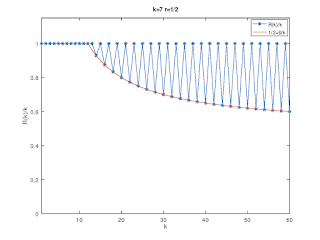Rank deficiencies for the k=7 r=1/2 mother code.Rank deficiencies for a k=7 r=2/3 code obtained by puncturing.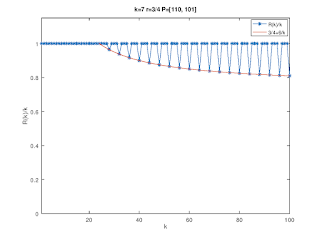Rank deficiencies for a k=7 r=3/4 code obtained by puncturing.Rank deficiencies for a k=7 r=4/5 code obtained by puncturing.

### MIL-STD-188-110D mini-probe base sequences

The mini-probes specified in MIL-STD-188-110D, Appendix D have interesting structures. Let us start with the two exceptions, $$n = 13$$ and $$n = 19$$:

### $$n = 13$$: (TABLE D-XXII)

This is the well-known Barker-13 sequence, i.e., \begin{equation} [+1, +1, +1, +1, +1, −1, −1, +1, +1, −1, +1, −1, +1]\;. \end{equation}

### $$n = 19$$: (TABLE D-XXIV)

This sequence is based on Legendre-19: \begin{equation} [1,\, -\mathsf{Legendre}(k/19)]\;. \end{equation}

### $$n = m^2$$: (TABLES D-XXIII, D-XXV - D-XXXVI)

All other mini-probe base sequences have lengths $$n=m^2$$ for $$4\le m \le 17$$ and can be obtained from the following formula, \begin{equation} MP(k;m) = \exp\left\{-2\pi i m \left\lfloor{\frac{k}{m}}\right\rfloor \frac{k}{n} \right\}\;, \end{equation} where $$\left\lfloor{x}\right \rfloor$$ denotes floor function which returns the greatest integer $$\leq x$$. Curiously, the mini-probe base sequences for $$m\ge 14$$ are the complex conjugate of the formula above, so I wonder if this is by design or it is an error in the standard.

Note that $$MP(k;4)$$ is the (complex conjugate of the) length-16 Frank-Heimiller sequence contained in TABLE C-VIII, i.e., \begin{equation} \exp\big\{2\pi i\, [0, 0, 0, 0,\; 0, 2, 4, 6,\; 0, 4, 0, 4,\; 0, 6, 4, 2]/8\big\}\;. \end{equation} Many pages could have been saved by describing the mini-probe base sequences in terms of the formula above.

## Tuesday, March 26, 2019

### 8-ary constellation bursts at 12800bps data rate

For background it might be helpful to read the relevant entries in i56578's blog.

Two consecutive bursts centered on 2670 kHz are shown below in terms of 287-symbol long frames. The preamble and mini-probes have been descrambled (gr-digitalhf) and therefore have phases close to 0 rad. There are 13 frames with payload data per burst, each one having 256 symbols/frame: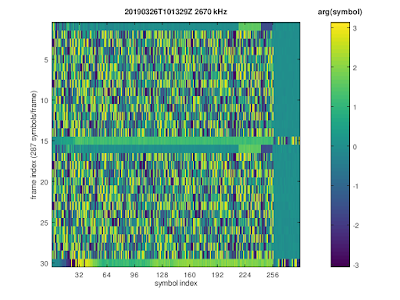Symbols after adaptive filtering and descrambling of MIL-STD-110C known symbols for consecutive bursts

These signals are perfectly compatible standard MIL-STD-110C for 12,800 bps data rate; note that for 12,800 bps there are no requirements on the interleaver and on the error coding specified. As was observed before, only 8 points out of the QAM-64 constellation are used.

Looking closer at the payload, a periodicity of 160 symbols was found. In terms of 160-symbol long frames the payload is shown below:Payload in terms of 160 symbol frames.
The fact that (symbol2) above is the same for all frames indicates that the payload consists of BPSK-modulated data which is scrambled to the 8PSK-like constellation. So what is left is to determine the scrambling.

It turns out that the used scrambling sequence is based on one of the scrambling sequences specified in STANAG 4538, i.e., it can be obtained as an extrapolation of one of the S4538 scrambling sequences: writing the 3-bit scrambling sequence in binary notation as PN(i) = bA(i)B(i)C(i), one can verify that A(i), B(i), and C(i) are subsequences of the same pseudo-random binary sequence generated by a certain LFSR. Determining the LFSR and the offsets of the subsequences is left as an exercise for the interested reader.

Once the payload symbols are descrambled, it becomes apparent that they consist of 104 Walsh-modulated di-bits (104×32 = 13×256) where each 4-symbol long Walsh symbol is repeated 8 times:Descrambled payload revealing 104 Walsh-coded di-bits.

## Sunday, March 24, 2019

### Interesting MSK-modulated signals on HF (2)

This is an update of the last blog post.

Yesterday, another signal was picked up for which both the "X" and the "Y" bit streams were generated by the same LFSR with polynomial [3,1,0] × [17,16,15,14,13,12,10,8,5,4,0] and period N= (23-1)×(217-1)=917,497. The cross-correlation between "X" and "Y" show that the "X" bit stream is offset w.r.t. "Y" bit stream by M=(N-1)/2=458,748 bits.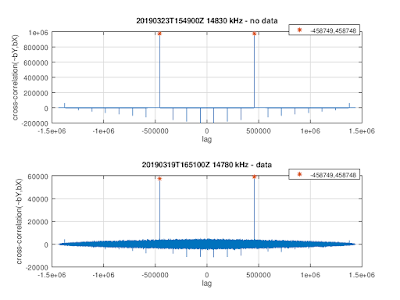"X" and "Y" bit stream cross-correlations

The data in the "X" channel can descrambled as follows
b(i) = XOR(~Y(M+i), X(i)),
and the auto-correlation of the descrambled bits b(i) has peaks at multiples of 48 bits: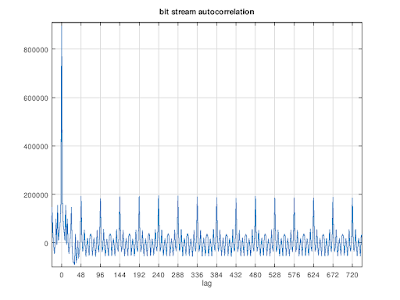Autocorrelation of the descrambled bit stream
In terms of 240 bit frames the descrambled bits look like this:Descrambled bit stream in terms of 240-bit frames

and in terms of 48-bit frames:Descrambled bit stream in terms of 48-bit frames
Here one can see that the descrambled bits come in pairs
b(2i) = b(2i+1)
so there are 24 pairs of bits per 48-bit frame. These 24 bits are not independent: they are determined by 6 bits only:
1  ~3    4  6 13  ~20
2   9  ~15
5  18 -~21
7   8   11 16 19 -~23
10  17
12  14 -~22
where ~P denotes logical NOT of bit number P and -P denotes a bit at position P from the previous frame.

These 6 bits are likely not independent. Interpreting them as a 6-bit binary number, the histogram of these numbers shows that they are not equally probable:Histogram of 6-bit frame interpreted as 6-bit binary numbers

## Thursday, March 21, 2019

### Interesting MSK-modulated signals on HF

Recently, a number of MSK-modulated signals with bandwidth ≈48 kHz were picked up on various KiwiSDRs on frequencies including 6840, 7730, 9490, 10640, 10840, 14730, 14780, and 14830 kHz. For some of these signals a TDoA analysis has been performed, pointing to a location close to Chicago:TDoA
Since the bandwidth of theses signals exceeds the available bandwidth of a single KiwiSDR channel, three recordings spaced 15 kHz were coherently combined using gr-kiwisdr.

The power spectrum of FFT(IQ2) shows two clear peaks at ±24kHz, so these signals are MSK modulated with 48,000 baud data rate.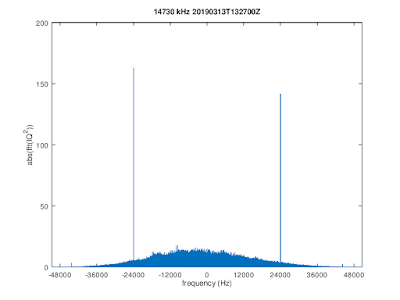FFT(IQ2) showing two clear peaks at ±baud/2 → MSK modulation

For the coherently combined recording, the MSK demodulation quality plots shown below indicate that the quality is sufficient for extracting bit streams. These and the following plots were made with Octave code based on signal-analysis.MSK demodulation quality plots

Using a coherent demodulation technique the "X" and "Y" bit streams were obtained, where the "X" bits are modulated onto cos(t) and "Y" onto sin(t). The data rate for both "X" and "Y" is 24,000 bits/sec.

The autocorrelations of the "X" and "Y" bit streams do not reveal any frame structure, i.e., "X" and "Y" look like streams of perfect random bits. However, it turns out that the "Y" bit stream is entirely made up by a pseudo-random sequence generated by a LFSR, so this presumably is how the start locations of frames can be recovered from the received signal.

The taps for the length-20 LFSR generating the "Y" bit stream are
T = ,
where the LFSR taps T are defined such that the following property holds for a given bit sequence b
b(i) == mod(sum(b(i-20:i-1) .* T), 2)

The period of this LFSR was determined by simulating 2M bits using the found taps and a start state taken from the data. It is N = 917,497 = (23-1)×(217-1). The fact that N factorizes indicates that the polynomial in GF(2) defined by T is not prime, and indeed:
[20,19,17,16,15,13,12,10,9,7,6,4,3,1,0] = [3,1,0] × [17,16,15,14,13,12,10,8,5,4,0].
A valid start state S which generates "Y" is
S = [0,0,0,0,0,0,0,0,0,0,0,0,0,0,0,0,0,0,0,1].The ~"Y" bit stream is generated by a LFSR with 20 taps.

No structures have yet been found for the "X" bit stream which presumably carries data.

## Saturday, March 16, 2019

### KiwiSDR IQ data streams with >20.25 kHz bandwidth (5)

By now, gr-kiwisdr can coherently combine between 2 and 6 IQ data streams recorded from the same KiwiSDR.

Following an idea by WA2ZKD, the following test was made with DRM signals: three data streams were recorded, one centered on the DRM signal on 15120 kHz and two more centered on 15112.5 kHz and on 15127.5 kHz which were then coherently combined into a signal data stream. The GNURadio display is shown below: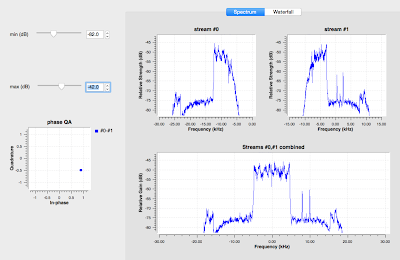Coherent combination of two IQ data streams using gr-kiwisdr.

Both, the WAV file centered on 15120 kHz, and the combined WAV file were successfully decoded by DREAM:

The SNR is comparable for both files, taking into account that it fluctuates by about ±0.5 dB:DREAM waterfall display for the combined WAV file.DREAM waterfall display for the WAV file recorded on the center frequency.

Only one slight difference was found in the SNR per carrier display: although the SNR per carrier is fluctuating quite a lot, for the combined WAV file there is a dip around the center which is not there for the WAV file recorded on the center frequency: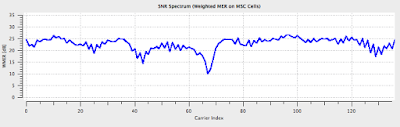DREAM SNR per carrier display display for the combined WAV file.DREAM SNR per carrier display for the WAV file recorded on the center frequency.

## Wednesday, March 6, 2019

### KiwiSDR IQ data streams with >20.25 kHz bandwidth (4)

This is a follow-up to this blog post.

The figure below summarizes how three IQ data streams with equal frequency offsets Δf are combined into a single IQ data stream with sampling frequency 4Δf: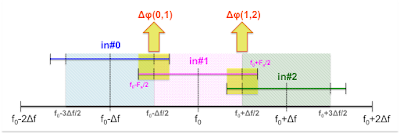Coherent combination of three KiwiSDR IQ streams

Note that the center frequencies are set to exact values, using GNSS timestamps to correct the local KiwiSDR oscillator, while the sampling frequencies of the IQ data streams are derived from the local KiwiSDR oscillator and are not exact. As a consequence the three IQ data streams are not coherent.

The three IQ data streams can be made coherent by 1) correcting for the frequency offsets and 2) aligning the relative phases.

### 1) Correcting for the frequency offset

One way of describing this correction is by comparing a signal in stream#1 with frequency ΔF/2  and another signal in stream#2 with frequency -ΔF/2, taking into account that there are two different sampling rates: the true (GNSS aligned) sampling rate Fs and the sampling rate according to the state of the local KiwiSDR oscillator, F′:
z1(n) = exp{2πinΔF/2F′s}
z2(n) = exp{2πinΔF/Fs - 2πinΔF/2F′s} .
The beat offset signal is given by
z1*(n) z2(n) = exp{2πinΔF(1/Fs - 1/F′s)} ,
and is used to correct for the frequency offset, where F′s is determined from the GNSS time tags in the KiwiSDR IQ streams.

### 2) Relative phase alignment

Having corrected the frequency offsets, we are left with constant relative phase differences, Δϕ(0,1) and Δϕ(1,2). These global phase offsets are estimated by cross-correlating the overlapping parts of the spectra, indicated in yellow in the figure above. GNURadio makes it easy to do this, using a combination of freq_xlating_ccf and conjugate__cc and a simple block which estimates the phase difference between two vectors of IQ samples.

Because the overlaps between IQ streams are needed to estimate the phase offsets, recordings with kiwirecorder.py should use the full available bandwidth.

The method described above has been implemented using GNURadio and is available as part of gr-kiwisdr. Please note that this is work in progress and might need further improvements.

As can be seen in the updated GRC flowgraph below, IQ stream sample alignment, the correction for coherence, and the PFB synthesizer were combined into a single GNURadio block, called coh_stream_synth. In addition, exp{iΔϕ(0,1)} and exp{iΔϕ(1,2)} are shown in a constellation diagram display in order to monitor phase coherence (=stable relative phases).GRC flowgraph

Using the GNURadio PFB synthesizer with 2× oversampling (twox=True), edge effects at the boundaries between IQ data streams are avoided: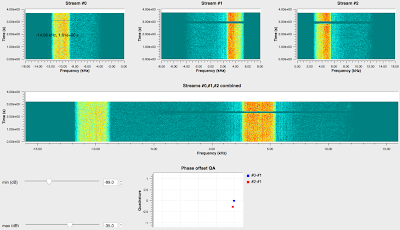Coherent combination of three IQ streams @12 kHz into a single IQ stream @32 kHz.

## Monday, February 18, 2019

### WBHF (1)

Below are a 16QAM and 32QAM constellation diagrams picked up from a KiwiSDR in ECNA: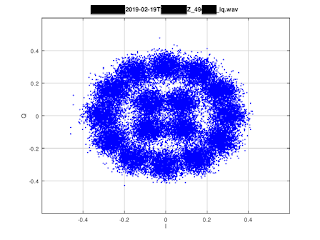16QAM constellation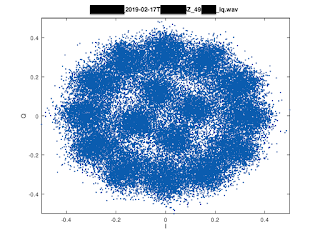16QAM constellation diagram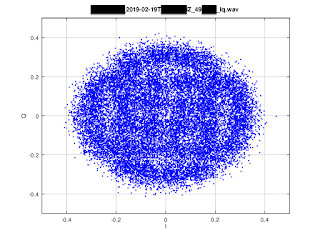32QAM constellation

All processing, i.e, symbol timing alignment and doppler offset correction, were done manually in GNU Octave.

## Tuesday, February 12, 2019

### KiwiSDR IQ data streams with >20.25 kHz bandwidth (3)

This is a follow-up to this recent blog post. In order to further verify the method of combining KiwiSDR IQ streams described before, an extreme example was considered: a STANAG 4285 signal centered on the border between two streams, see below.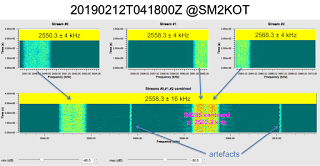Combination of three KiwiSDR IQ streams @8kHz into one @32kHz.

As before IQ wav files with GNSS timestamps were recorded using kiwirecorder.py on three center frequencies: f0-8kHz, f0, f0+8kHz. While the results obtained before using CODAR and other FMCW radar signals were promising, the fist results obtained with unmodified code were not satisfactory: the frame structure of STANAG 4285 was clearly visible when looking at abs(IQ), but the obtained constellation diagram was not in a good shape and it looked like there was a time-varying phase offset between the three input streams.

Note that the sampling rate of IQ samples from KiwiSDRs is eventually derived from a Quartz oscillator which is subject to temperature drift, so the actual sampling rate in the example shown here is 12001.18217 Hz instead of 12000 Hz. While the local oscillator center frequencies are set taking into account the deviation of the local oscillator (using GNSS position+time solutions) and are exact, the sampling frequency is not. Therefore streams #2 and #0 had to be shifted in frequency by ±8000 Hz × (12001.18217/12000-1) = ± 0.7881 Hz (this is a phase shift of  ±284 degrees per second) in order to avoid "frequency overlap" and this solved the problem. Thanks to GNU Radio shifting streams of IQ samples in frequency takes only a few clicks in gnuradio-companion.

Below symbol phases vs. symbol number and the constellation diagram are shown for a time interval of 2.5 seconds while ionospheric propagation was stable. For this all processing was done using GNU Octave, manually correcting for doppler offset and symbol timing, i.e., no adaptive filter was used.STANAG4285 symbol phase vs. symbol number.IQ display for the STANAG 4285 signal integrated over 2.5 seconds.

While all this looks promising more tests are needed.

## Tuesday, February 5, 2019

### Chirp sounder measurements with KiwiSDRs (1)

The plots below show measurements of the chirp sounders from Cyprus (RAF Aktrotiri). There are two chirps (100kHz/sec) separated by 5 seconds and repeating each 5 minutes. In G3PLX notation they are called 300:240 and 300:245

Using GNSS timestamped IQ samples from the KiwiSDR @IU8CRI, tuned to 13,200 kHz, a pair of chirps was analyzed in terms of instantaneous frequency and of propagation time delays obtained from the FFT of the de-chirped signals.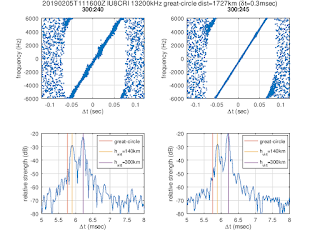Top: instantaneous frequency; bottom: propagation time delay obtained form the FFT of the de-chirped signal

In this earlier blog post a time offset of about δt=0.3±0.03 msec w.r.t. start time at the beginning of a given UTC second of the chirp signals from Cyprus was found in the chirp monitoring data from the U Twente WebSDR. This offset is also found in the KiwiSDR measurements, i.e., without applying this offset, the first peak in the propagation time delays would be below the equivalent time of propagation along the great-circle distance at the speed of light.

It would be very interesting to implement a more systematic monitoring of chirp sounders using KiwiSDRs.